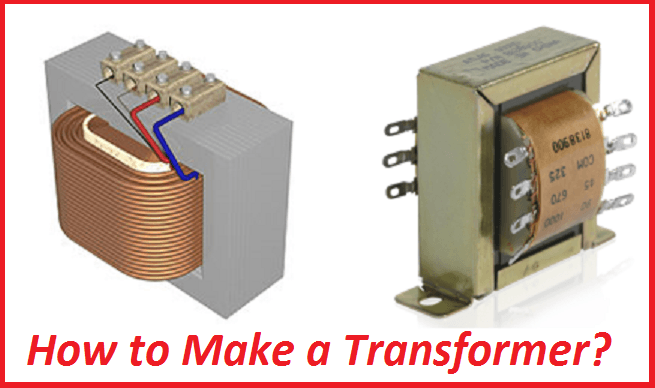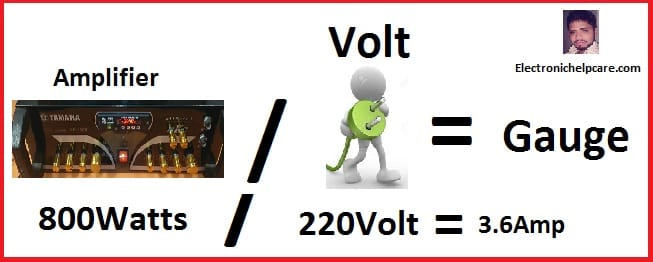Breaking News

# how to make transformer

## how to make a transformer.

first, we need to know to make construct a transformer. To build it we need to know where we are going to use the transformer. about Voltage, Watts, and ampere need. Before all, we must know about the wire gauge and ampere. we are going to construct.

Constructing:  to calculate the wire ampere?AWG Gauge     –      Ampere

7  AWG            –        55.0

8  AWG            –        50.0

9  AWG            –        57.5

10 AWG           –        35.0

11 AWG           –        30.0

12 AWG           –        25.0

13  AWG          –        22.5

14 AWG           –        20.0

15  AWG          –          18.5

16 AWG           –         17.0

17  AWG          –          15.5

18  AWG          –          14.0

19  AWG          –          12.5

20  AWG          –          11.0

21  AWG          –          8.0

22 AWG           –          7.0

23 AWG           –          6.0

24 AWG           –          3.5

25  AWG           –          3.0

26  AWG          –          2.2

27  AWG          –          2.0

28  AWG           –          1.4

29  AWG          –          1.20

30  AWG          –          0.86

to calculate the wire ampere?

First, we have to decide where we are going to use it.

Watts, do we need it?

If we want to make an 800 Watts amplifier. Then we need 800 Watts.

## First for the primary binding

We know that,Watts / AC Voltage=Ampere.

So 800watts/220voltage=3.6amp

The 3.6-ampere wire we need for the primary wire

we can see the list, 18 number wire is 3.2 amperes and 17 number wire is 4.1 amperes. But we need 3.6 amperes.

We can use 17 numbers for good performance.

## 2nd for the secondary binding

We need 800 Watts

What voltage and ampere do we need?

we know Voltage X Ampere = Watts.

If we use 50 Voltage So how many Amperes do we need?

Amperes?

800/50=16 Ampere Gauge Wire.

We need 16 amperes Gauge wire.

According to our Gauge List

11 number Wire is 16.6 Amperes

So we have to use 11number gauge wire for the secondary Binding.

here have a video of the binding it. we can calculate the core area and also can calculate how many turns need for binding it. and how to build it. if you watch the video you can learn. but this is small. don’t worry all transformer are the same rules to make.

Now lets Go to Watch the video,

## construct a transformer?

transistor circuit diagram of 2sa1943 and 2sc5200

Thanks a lot to be with us. If you want another post then please visit our website.

We have another post for you. like repairing amplifier,

Please like comment and share our post.